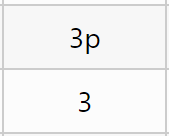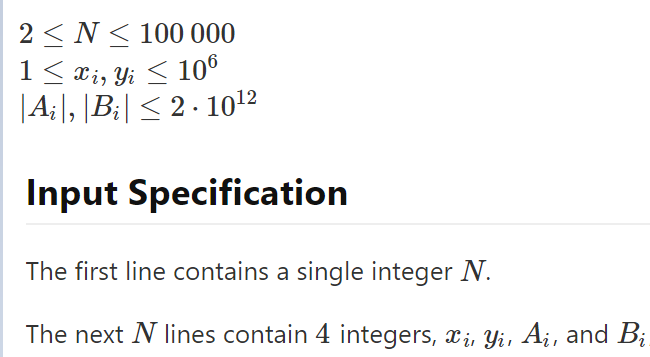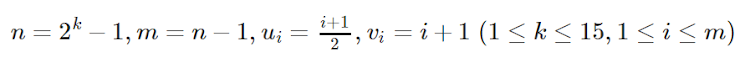Jekyll2023-06-14T06:44:57+00:00http://0.0.0.0:8000/feed.xmlMoses’ WebsiteMy Personal WebsiteMoses Xumoses@mosesxu.netPhones and Markov Chains2021-12-27T21:45:00+00:002021-12-27T21:45:00+00:00http://0.0.0.0:8000/contest/math/phones-and-markov-chains MathJax.Hub.Config({ TeX: { equationNumbers: { autoNumber: "AMS" } } });

Around four months ago, I competed in the Noctem Virtual II (Div. 1), where I encounted a very interesting expected value problem.

The statement is as follows:

You are given two arrays of integers $$A$$ and $$B$$ (where $$|A|=|B|,0 \le A_i,B_i \le 9$$), along with an integer $$K\ (2 \le K \le 10)$$, and you want to transform $$A$$ into $$B$$. However, the only operation you can perform is the following:

Pick a random index $$1 \le i \le |A|$$ where $$A_i \neq B_i$$, and change $$A_i$$ to one of $$\{A_i, A_i+1, \ldots, A_i+K-1\}$$ uniformly randomly. Note that if a value ever goes above $$9$$, it loops back to $$0$$.

Your goal is to compute the expected number of operations to transform $$A$$ into $$B$$.

 Constraints: $$1 \le A \le 10^5$$

The first thing you may notice is that operations are independent between digits, meaning that all you really need to do is to calculate the expected number of operations per digit and just sum those together. This lets us focus on the case where $$|A|=|B|=1$$, which is the only one that really matters.

There are many ways to approach this problem, but one that I found quite interesting (and the one that we did in-contest) was to think of the current value of $$A_1$$ as a state, and the operation on each digit $$A_1$$ can take on as transitions to other states (each with probabilities). In other words, a Markov chain!

If we let $$P(i)$$ denote the probability $$A_1$$ becomes $$B_1$$ after exactly $$i$$ operations, our answer is:

$\sum_{i=0}^\infty iP(i)$

Observe that our system is an Absorbing Markov chain, which tells us that $$\lim\limits_{n \to \infty} P(n) = 0$$ since eventually every system ends up at the state $$B_1$$, and quite quickly too, since the lowest probability of a transition is only $$10\%$$. Thus, if we just wanted to approximate the answer to a sufficiently precise value, we could use a simple dynamic programming to compute $$P(1), P(2), \ldots, P(k)$$ for some large enough $$k$$, and we would be done. Unfortunately, the original problem asks us to output the answer modulo a large prime, so this approach would not work as we need the exact value.

For the full solution, we need to try something else. Let’s first try and rephrase our formula from earlier. Let $$Q(i)$$ be the probability that we need to take at least $$i$$ operations to reach $$B_1$$. Notice that $$Q(i) = 1 - \sum_{j=0}^{i-1} P(j) = \sum_{j=i}^\infty P(j)$$ since $$\sum_{i=0}^\infty P(i) = 1$$. This then gives us the following expression

$\sum_{i=0}^\infty iP(i) = \sum_{i=1}^\infty Q(i)$

since you may notice that by summing all $$Q(i)$$, $$P(1)$$ is counted once, $$P(2)$$ is counted twice, $$P(3)$$ is counted three times, etc.

Next, let $$M$$ denote the matrix of transitions for the system. In particular, $$M_{i,j} = 1/K$$ if $$i \neq B_1$$ and there exists $$0 \le x < k$$ such that $$i + x \equiv j \pmod{10}$$, and is $$0$$ otherwise.

Lastly, let the vector $$\alpha$$ represent the initial state of our system, so $$\alpha_i = \begin{cases} 1 & i = A_1 \\ 0 & i \neq A_1 \end{cases}$$.

Let $$\text{vsum}(v) = \sum_{i=0}^9 v_i$$. Notice that if we multiply a state vector by $$M$$, it effectively preforms the operation on every possible state of the system that the vector represents. Additionally, if there was a possibility that the system was in the state $$B_1$$, that probability “exits” the system. Mathematically, this means that $$\text{vsum}(Mv) = \text{vsum}(v) - v_{B_1}$$ for all vectors $$v$$. This combined with the notion that $$(M^k\alpha)_i$$ denotes the probability that the system is in state $$i$$ after $$k$$ operations allows us to deduce that $$Q(k) = \text{vsum}(M^k\alpha)$$.

We now get:

$\sum_{i=1}^\infty Q(i) = \sum_{i=1}^\infty \text{vsum}(M^k\alpha)$

and since $$\text{vsum}(v + w) = \text{vsum}(v) + \text{vsum}(w)$$,

$= \text{vsum}\left( \sum_{i=1}^\infty M^k\alpha \right)$ $= \text{vsum}\left( \alpha \left( \sum_{i=1}^\infty M^k \right) \right)$

Now, to the last and most interesting part of the solution: notice that the sum with $$M^k$$ looks awfully like a geometric series- because it is! Additionally, since we’re working in a matrix field, we’re actually able to just use the formula for the sum of an infinite geometric series to find the sum!

$= \text{vsum}\left( \frac M{I - M} \alpha \right)$

I’m not sure on the specifics of why this works, since the proof for the formula is already quite involved for just reals, but we can build some intuition on when/why this works by looking at other number systems. My thoughts are that other than commutativity, (in this case, real-valued) matrices share many algebraic properties with the real numbers which means that they’ll behave nicely with a lot of theorems that we expect from the real numbers, either directly or with a bit of generalizing.

As for when this formula applies to matrices $$M$$, if we can expect for the sum $$M + M^2 + M^3 + \ldots$$ to converge, then the formula should work. For example, other Absorbing Markov chains.

]]>
Moses Xumoses@mosesxu.net
DMOJ Problemsetter Quiz Guide2021-12-27T20:19:00+00:002021-12-27T20:19:00+00:00http://0.0.0.0:8000/contest/dmoj-problemsetter-quiz MathJax.Hub.Config({ TeX: { equationNumbers: { autoNumber: "AMS" } } });

This post is pretty straightforward- it’s just a guide to the questions on the DMOJ problemsetter quiz for those that need a bit of a hand :)

Where possible, I try to link to the relevant documentation.

First and foremost, the problem setter quiz is here

Now, let’s go over the questions:

Email

DMOJ Handle

😛

What should you put in the field if you wanted a memory limit of 512 MB?

Memory limits are given in Kilobytes.

What does enabling short circuit do?

If you’re familiar with problems that use subtasks, short-circuiting will terminate judging if a subtask fails.

What is the difference (permission-wise) between a creator, curator, and a tester?

Relevant documentation here.

What is the difference between the points for these two problems?p is for partial points!

What format does DMOJ use for its problem statements and math equation rendering?

I’m not sure about the exact name, but it’s just Latex. If you’re familiar with Polygon, it’s almost the same system except ~ are used instead of \$ to denote inline math.

How would you ensure your data is correct for the following input specification using asserts? (Write out a working program using the language of your choice)If you want a bit more context on the problem, this is the problem that the question uses. Other than that, the question is pretty straightforward- you just write a program to read the input and check if all the constraints are satisfied using assertions.

Note that you don’t have to check for formatting for this question, just that the contraints are satisfied.

Should input and output data end with a newline?

If you don’t do this I’ll eat all of your food.

How would you type out the following expression in a problem statement?Remember how DMOJ uses latex?

Here’s a little practice website if you’re interested :)

When using a generator to create test data, what does the output stream and error stream represent, respectively?

Relevant documentation here.

What is the difference between these checkers: an absolute floating point error and a relative floating point error?

Make sure to read up on approximation error, though you should avoid using floating-point checkers when possible, since the innacuracy isn’t fun.

Where can you find problem examples utilizing different graders?

Relevant documentation here.

True or False: Checking the “Pretest?” box in the Edit Test Data page will mark the case as a sample case.

This question is a bit of a joke that comes from some recent DMOJ lore. If you’re familiar with pretest/systest platforms such as Codeforces, you should be able to answer this from common sense alone.

Using your preferred language, print an array of integers called “arr” on a single line, space separated, and to standard error.

Again, a pretty straightforward programming task. There isn’t much to say here.

What is the difference between the output prefix length and the output limit length?

Try submitting something incorrect on DMOJ and you may notice that the judge lets you see part of your output- this is the output prefix length. The output limit length is just the maximum output size the judge will accept. This is especially useful when using custom checkers so that they don’t try and read an output that’s too large.

Is setting a zero point value for a case legal?

Hint: Sample test cases

Fill in the blank: Generators __ have a fixed seed.

I’ll answer this with an example: if you were debugging a generator, would you like if it behaved differently every time it ran?

What is the difference between the line-by-line checker and the unordered checker?

Make sure to read up on your checkers! However, you won’t be seeing these two checkers often though.

Fill in the blank: You should use __ media uploader for problem statements.

The DMOJ site has a media uploader for images and other files in problem statements, use it!

I hope this was useful!

]]>
Moses Xumoses@mosesxu.net
Lazy Divide and Conquer2021-08-20T04:02:00+00:002021-08-20T04:02:00+00:00http://0.0.0.0:8000/contest/lazy-cdq MathJax.Hub.Config({ TeX: { equationNumbers: { autoNumber: "AMS" } } });

Hi all!

Today I’ll be presenting a simple, but interesting Divide and Conquer trick that lets you process 2D range updates and range queries quickly and with low memory usage.

The basis of this form of Divide and Conquer is the segment tree, except instead of being used to process queries and updates online, we’ll be traversing the segment tree im a way similar to a BFS or DFS search. This allows us to use a 1D data structure to compute the answers to each query. This usually results in an improved memory complexity (usually by a log factor) along with a smaller hidden constant in the time complexity (which I believe is due to better cache locality).

To motivate the algorithm, I’ll present a sample problem:

Given an $$N \times N$$ grid $$A$$ (all initially zeros), process $$Q$$ operations of the following types:

1. Given the integers $$l,r,d,u,v$$, assign $$A_{i,j} := A_{i,j} + v$$ for all $$l \le i \le r, d \le j \le u$$
2. Given the integers $$l,r,d,u$$, output the value $$\sum_{i=l}^r \sum_{j=d}^u A_{i,j}$$

Note that this question is simply asking to support 2 types of operations on a 2D grid: 2D range increment and 2D range sum. From now on, the problem will be referred to in this context.

Additionally, let’s assume that $$l=r$$ and $$d=u$$ for every update, so we don’t need to worry about range increments. Then, after we solve this sub-problem, we’ll generalize our solution to work for any $$l,r$$ and $$d,u$$.Our solution to the sub-problem works by performing Divide and Conquer on the first dimension (l-r), and then using a segment tree (fenwick tree works too in this case) to perform range queries across the second dimension (d-u).

We start by considering the entire range of values across the first dimension (setting $$l=1,r=N$$) and all operations. We then apply all the operations in order, applying all updates but only computing queries which occupy the whole l-r range. Note that this means we effectively treat each operation as 1-dimensional, which means we only need to consider the d-u dimension of each operation.

Finally, we divide our range into two halves, and then propagate all queries and updates to a half if it partially or completely covers it, but not if it covers the whole l-r range already.

Below is some pseudocode, which some may find more helpful than the wordy explanation:

solve(l, r, operations):  # Call solve(1, N, operations) to compute all answers
covers(op):
return op.l <= l and r <= op.r

reset_segment_tree()
for op in operations:
if op is update:
update_segment_tree(op.d, op.v)
else if op is query and covers(op):
ans[op.index] += query_segment_tree(op.d, op.u)

left = []
right = []
mid = (l + r) / 2
for op in operations:
if not covers(op):
if op.l <= mid:
left.append(op)
if op.r > mid:
right.append(op)

solve(l, mid, left)
solve(mid+1, r, right)


# Complexity Analysis

In our Divide and Conquer, each update is either pushed to the left or right (and never both) recursive call. And as the recursion will only ever go $$\mathcal{O}(\log{N})$$ layers deep, each update must be processed at most $$\mathcal{O}(\log{N})$$ times. For the queries, we can observe that for a given query $$l,r,d,u$$, the ranges that the query will be processed at are identical to the ranges considered by a 1D segment tree processing a range query from index $$l$$ to $$r$$. This means that each query will be processed at moat $$\mathcal{O}(\log{N})$$ times. Thus, the overall time complexity of the solution is $$\mathcal{O}(Q \log^2{N})$$

As for memory usage, the only memory we need to worry about is the segment tree and the list of operations held in the stack when performing the Divide and Conquer. The first source of memory usage clearly uses $$\mathcal{O}(N)$$ memory, but the second is a bit more complicated. Our worst case is when all of our operations are present at each level of recursion (i.e. $$l=r=1$$ for all operations), which would give us $$\mathcal{O}(Q \log{N})$$ operations being stored at once as there are $$\mathcal{O}(\log{N})$$ levels of recursion in our Divide and Conquer.

Thus, the total memory complexity should be $$\mathcal{O}(N + Q \log{N})$$.

Now, let’s generalize our updates to any $$l,r,d,u$$. You may think that this will make our code quite complicated, as it would involve some form of offline lazy propagation (given how our algorithm resembles updates and queries on segment trees). However, there already exists a powerful trick that lets us perform range updates on a segment tree without lazy propagation, and this is something we can adapt to our algorithm as well.

The only real issue preventing us from just propagating updates to both sides is that a single update covering the entire range 1-N in the 1st dimension will propagate to $$N$$ copies of itself by the bottom layer. Obviously, applying every single update $$N$$ times will not work, but there is a way to optimize: treating updates like queries.

If any update completely covers the range we’re currently on, we won’t propagate it further. Instead, we apply all the operations again, but only consider updates that completely cover the range and ALL queries (you may notice that this is the reverse of what we were doing earlier, where we only considered queries covering the range but ALL updates). Thus, the expanded pseudocode would be the following (note that new/changed lines are marked with a * at the end):

Note that this time, our segment tree also needs to support range increment.

solve(l, r, operations):  # Call solve(1, N, operations) to compute all answers
covers(op):
return op.l <= l and r <= op.r

reset_segment_tree()
for op in operations:
if op is update:
update_segment_tree(op.d, op.u, op.v)            *
else if op is query and covers(op):
ans[op.index] += query_segment_tree(op.d, op.u)

reset_segment_tree()                                 *
for op in operations:                                *
if op is query:                                    *
ans[op.index] += query_segment_tree(op.d, op.u)  *
else if op is update and covers(op):               *
update_segment_tree(op.d, op.u, op.v)            *

left = []
right = []
mid = (l + r) / 2
for op in operations:
if not covers(op):
if op.l <= mid:
left.append(op)
if op.r > mid:
right.append(op)

solve(l, mid, left)
solve(mid+1, r, right)


Lastly, if you are in need of some code and a sample problem, here is my solution to a problem that uses this trick. Note that this problem varies slightly from the given sample problem in that we only need to check if the sum of a subrectangle is $$>0$$, which also means that our lazy function can be slightly incorrect but still be accepted since the exact sum is not relevant to our answer.

# Extra Notes

The biggest note I have for the blog is (surprisingly enough) the hidden constant of the runtime. While in my experience, this trick is preferable to using 2D data structures such as sparse fenwick tree, 2D segment tree, and segment tree of binary search trees, it still has a large constant factor. This is because updates have be done and undone multiple times, which means your 1D data structure needs to be very efficient for a low runtime. This also means that for some coders who are very advanced with 2D data structures, this trick may not improve runtime.

Additionally, I want to note the motivation I had for this trick. The non-lazy version (where you only have to process point updates), is (to my understanding) a well-known trick first presented by CDQ in her China TST Paper from 2008. Meanwhile, the lazy version was just the result of me slapping together CDQ Divide and Conquer with some funny segment tree shenanigans :)

]]>
Moses Xumoses@mosesxu.net